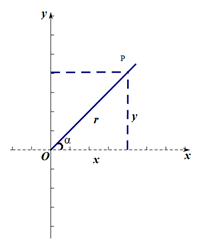# 角度与弧度

#### 常见的弧度：

360°=2π，180°=π，90°= π2 $\frac{π}{2}$，0°=0。

# 三角函数

#### 三角函数定义：#### 简单关系式：

sin²α + cos²α = 1

tan α = sinαcosα $\frac{sin α}{cos α}$

cos(α-β) = cosα·cosβ+sinα·sinβ

sin(α+β) = sinα·cosβ+cosα·sinβ

sin(α-β) = sinα·cosβ-cosα·sinβ

tan(α+β) = tanα+tanβ1tanαtanβ $\frac{tan α + tan β}{1 - tanα·tanβ}$

tan(α-β) = tanαtanβ1+tanαtanβ $\frac{tan α-tan β}{1+tanα·tanβ}$

sin2α = 2sinα·cosα

cos2α = cos²α-sin²α = 1-2sin²α = 2cos²α - 1

tan2α = 2tanα1tanα $\frac{2tanα}{1-tanα}$

cosα·cosβ = 12 $\frac{1}{2}$[cos(α+β)+cos(α-β)]

sinα·sinβ = 12 $\frac{1}{2}$[cos(α-β)-cos(α+β)]

sinα·cosβ = 12 $\frac{1}{2}$[sin(α+β)+sin(α-β)]

cosx+cosy = 2·cos x+y2 $\frac{x+y}{2}$·cos xy2 $\frac{x-y}{2}$

cosx-cosy = -2·sin x+y2 $\frac{x+y}{2}$·sin xy2 $\frac{x-y}{2}$

sinx+siny = 2·sin x+y2 $\frac{x+y}{2}$·cos xy2 $\frac{x-y}{2}$

sinx-siny = 2·cos x+y2 $\frac{x+y}{2}$·sin xy2 $\frac{x-y}{2}$

sin²α= 1cos2α2 $\frac{1-cos2α}{2}$

cos²α= 1+cos2α2 $\frac{1+cos2α}{2}$

03-161337
05-0609-05312
11-081767
10-27753
06-17185
07-215360
01-052150
03-31219